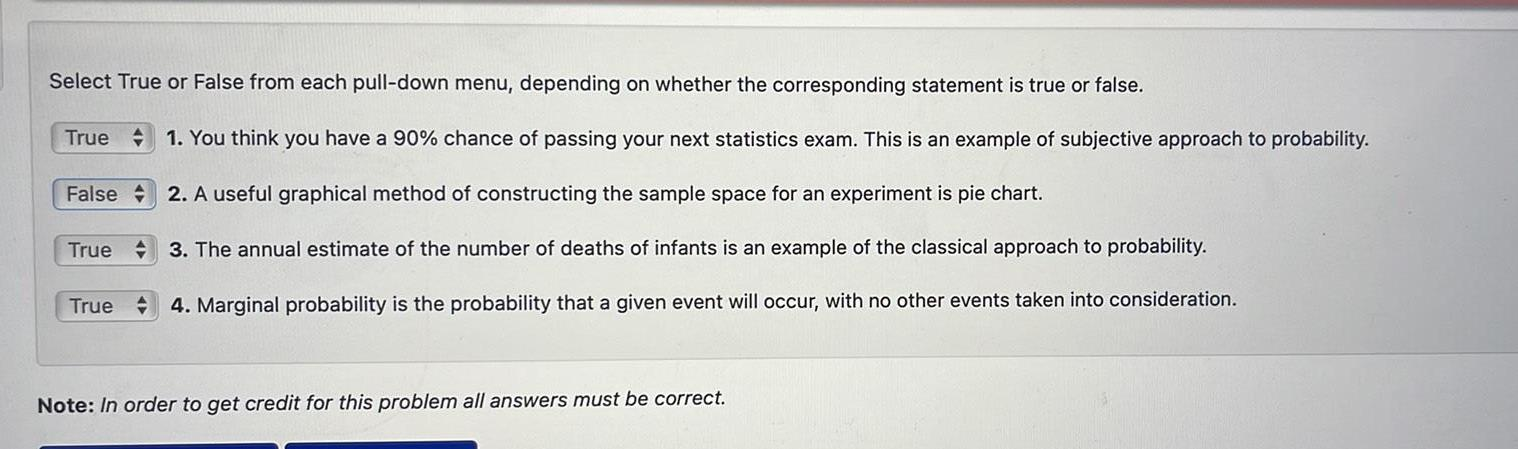Question:

# Select True or False from each pull down menu depending on

Last updated: 9/18/2023Select True or False from each pull down menu depending on whether the corresponding statement is true or false True 1 You think you have a 90 chance of passing your next statistics exam This is an example of subjective approach to probability 2 A useful graphical method of constructing the sample space for an experiment is pie chart 3 The annual estimate of the number of deaths of infants is an example of the classical approach to probability True 4 Marginal probability is the probability that a given event will occur with no other events taken into consideration False True Note In order to get credit for this problem all answers must be correct